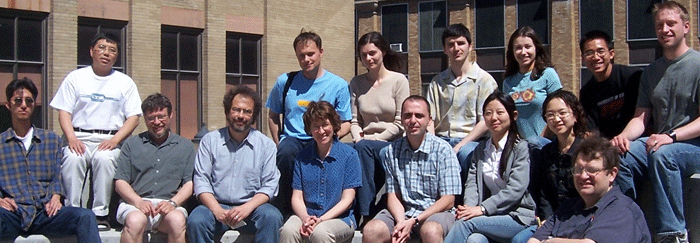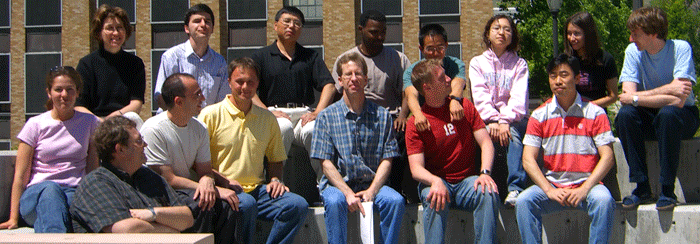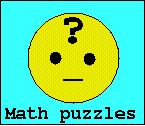Professor

#Department of Mathematics
University of Utah
155 South 1400 East, JWB 233
Salt Lake City, UT 84112-0090
Email: elena@math.utah.edu
Tel :  801 - 581 7315
Fax :  801 - 581 4148The mathematician is an artist whose medium is the mind and whose creations are ideas.
H.S.Wall, Creative Mathematics

 Research Areas:Inverse ProblemsNumerical MethodsOptimizationInhomogeneous mediaApplications: Materials, Geophysics, Biomechanics

Fractals - Undergraduate Colloquium, Spring 2012

Selected Papers
 Teaching:   Fall 2021 Math 5710  Introduction Applied Mathematics M W F / 11:50 AM - 12:50 PM LCB 121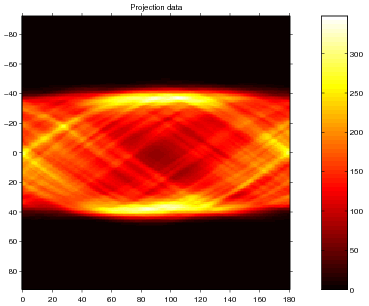Past Teaching
 Math 5750 /6880  Game Theory Math 5710  Introduction to Applied Mathematics   Math 5470 /6440 Chaos and Nonlinear systems    Computational Inverse Problems Math 5410 /6840  Ordinary Differential Equations Math 5740 Mathematical  Modeling   Math 2280  Differential Equations Math 3150 Partial Differential Equations Math 4950 /5910   Fractals Applied Complex Variables Math 5600 Survey Numerical Analysis Math 1210 Calculus I   Math 1100  Quantitative Analysis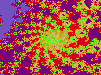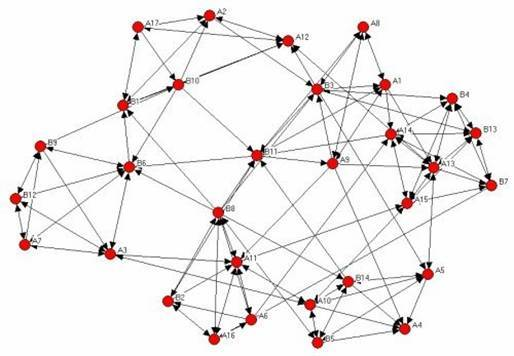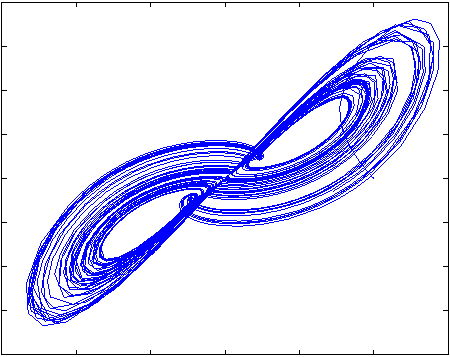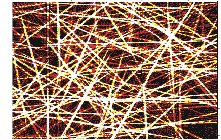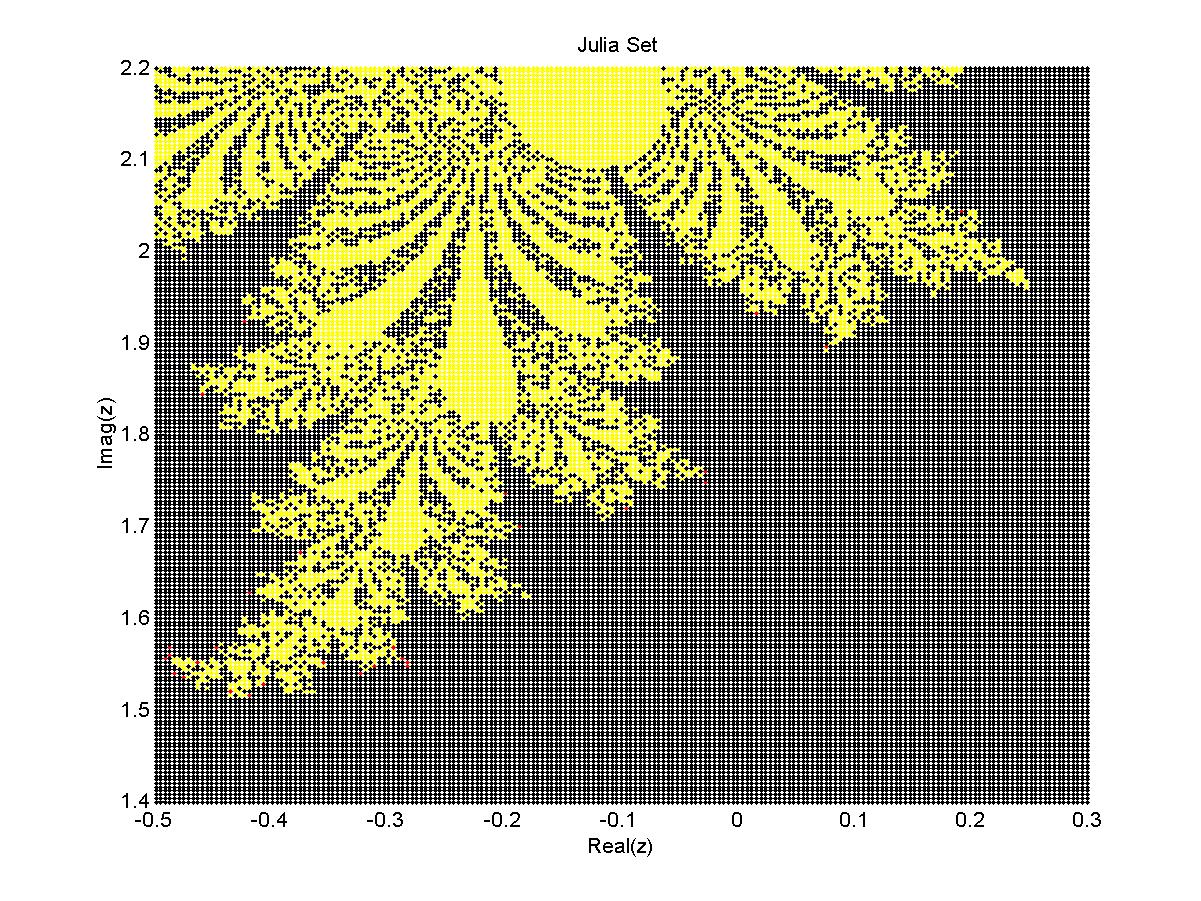Nonconvex Variational Problems - VIGRE Mini-course
May 16-27, 2005